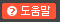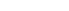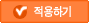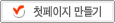# 태터데스크 관리자# 태터데스크 메시지

저장하였습니다.

## [Algorithm] ρ-approximation algorithm

Algorithm 2012. 2. 29. 10:47

This means that the approximation result f(x) should be between

a) ρOPT <= f(x) <= OPT, when ρ < 1
b) OPT <= f(x) <= ρOPT, when ρ > 1

So, a) occurs when it's a maximization problem, and b) occurs when it's a minimization problem.

For example of a) when ρ=0.5, and you want to find a 0.5-approximation algorithm for a NP maximization problem of a graph G=(V,E)

when, OPT = |E|

you need to find a approximation algorithm f(x) which finds at least

0.5|E| <= f(x) <= |E|

(for 0.5-approximation in a graph, randomized algorithm almost fits to the approximation with factor 0.5)

so, if ρ becomes closer to 1, it becomes a tighter approximation algorithm.

Fin.

#### 이 글을 공유하세요.

• . 2013.12.24 00:14

.